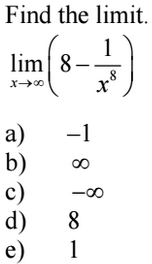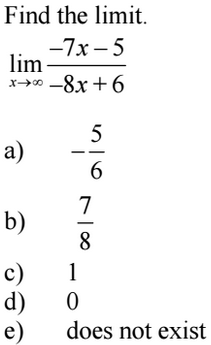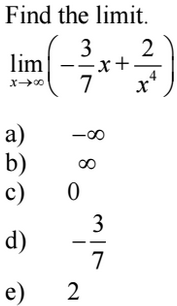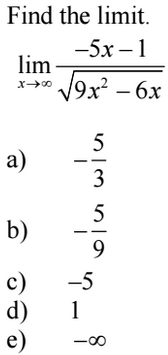### Day 44 - Limits at Infinity - 03.16.15

Update
• Unit 3 Test on 3/27!
• If you are leaving for spring break early, please make arrangements with me to take the test before break!

Questions

Bell Ringer
1. Limits at Infinity1. Limits at Infinity1. Limits at Infinity1. Limits at InfinityReview
• Extrema/Critical Numbers (video)
• How is the derivative used to locate the minimum and maximum values of a function on a closed interval?
• Find critical numbers using differentiation.
• Find extrema on a closed interval using differentiation.
• Rolle's Theorem (videoand Mean Value Theorem  (video)
• How are Rolle's Theorem and Mean Value Theorem related to differentiation?
• Apply understanding of Rolle’s Theorem and the Mean Value Theorem.
• Increasing/Decreasing Functions (video)
• How can the first derivative to determine whether a function is increasing or decreasing?
1. Determine intervals on which a function is increasing or decreasing.
2. Apply the First Derivative Test to find relative extrema of a function.
• Concavity and Inflection Points
• How can the second derivative be used to determine the concavity of intervals of a function? (video)
1. Determine intervals on which a function in concave upward or downward.
2. Apply the Second Derivative Test to find inflection points of a function.

Lesson
• Limits at Infinity (video)

Exit Ticket
• Posted on the board at the end of the block
Lesson Objective(s)
• How can limits be used to find horizontal asymptotes of the graph of a function? (video)
Skills
1. Determine horizontal asymptotes using limits.

#### In-Class Help Requests

Standard(s)
• APC.4
• Investigate asymptotic and unbounded behavior in functions.
• Includes:
• describing and understanding asymptotes in terms of graphical behavior and limits involving infinity
• APC.8
• Apply the derivative to solve problems.
• Includes:
• ​analysis of curves and the ideas of concavity and monotonicity
• optimization involving global and local extrema

Past Checkpoints